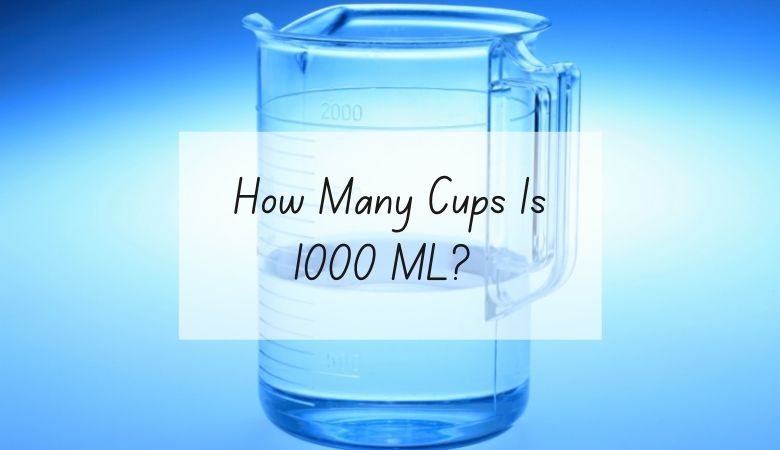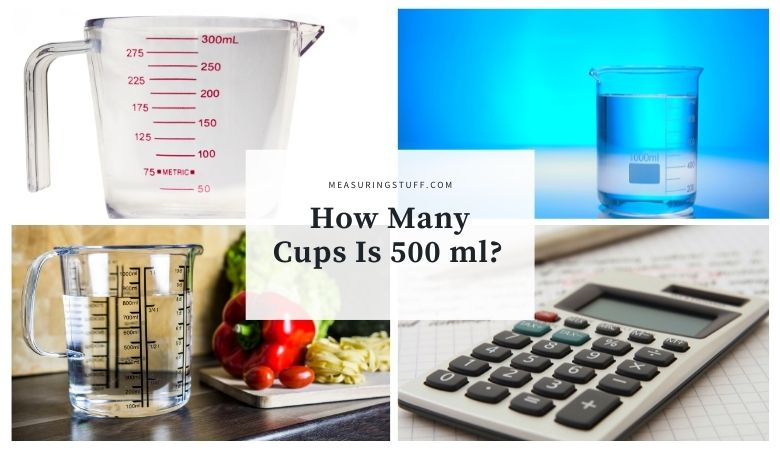# Volume## How Many Ounces Are In A Cup? (Exact Measurements)

Fluid ounces and cups are both units used to measure volume in the imperial system. Because this system doesn’t use a base-ten to calculate, it can be a little challenging to figure out how many ounces are in a cup. Fortunately, the math isn’t too difficult. There are 8 ounces in 1 cup. This means …## How Many Milliliters(ml) In A Gallon?

1 US Gallon equals 3785.41 milliliters. To convert US gallons into milliliters, you need to multiply by the conversion factor of 3785.41. For example, if you want to know how many milliliters are in 1 US gallon, multiply the number of gallons by 3785.41 to get the answer in milliliters. 1 US gallon x 3785.41 …## How Many Cups Is 1000 ML?

There are 4.22 cups in 1000 milliliters. One cup and 1000 milliliters are both common measurements you might encounter, particularly if you’re baking or cooking. Calculating how many cups are in 1000 milliliters can at first seem a bit tricky since these units are parts of two different measurement systems. However, with a bit of …## How Many Cups Is 500 ml?

There are 2.11 cups in 500 milliliters. One cup and 500 milliliters are both common measurements you might encounter, particularly if you’re baking or cooking. Calculating how many cups are in 500 milliliters can at first seem a bit tricky since these units are parts of two different measurement systems. However, with a bit of …## How Many Liters In A Gallon?

Liters and gallons are similar units of measurement in that they’re both designed to measure volume. However, these units of volume are from different measurement systems, so converting between the two can sometimes seem like a challenge. Luckily, there is a simple formula that can help you quickly figure out how many liters are in …